# Functions¶

## Sources¶

This lesson is partly based on the Software Carpentry group’s lessons on Programming with Python.

## What is a function?¶

A function is a block of organized, reusable code that can make your scripts more effective, easier to read, and simple to manage. You can think functions as little self-contained programs that can perform a specific task which you can use repeatedly in your code. One of the basic principles in good programming is “do not to repeat yourself”. In other words, you should avoid having duplicate lines of code in your scripts. Functions are a good way to avoid such situations and they can save you a lot of time and effort as you don’t need to tell the computer repeatedly what to do every time it does a common task, such as converting temperatures from Fahrenheit to Celsius. During the course we have already used some functions such as the print() command which is actually a built-in function in Python.

## Anatomy of a function¶

Let’s consider the task from the first lesson when we converted temperatures from Celsius to Fahrenheit. Such an operation is a fairly common task when dealing with temperature data. Thus we might need to repeat such calculations quite frequently when analysing or comparing weather or climate data between the US and Europe, for example.

### Our first function (aww…)¶

Let’s define our first function called celsiusToFahr.

:

def celsiusToFahr(tempCelsius):
return 9/5 * tempCelsius + 32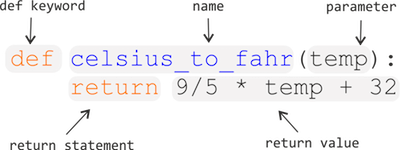The function definition opens with the keyword def followed by the name of the function and a list of parameter names in parentheses. The body of the function — the statements that are executed when it runs — is indented below the definition line.

When we call the function, the values we pass to it are assigned to the corresponding parameter variables so that we can use them inside the function (e.g., the variable tempCelsius in this function example). Inside the function, we use a return statement to define the value that should be given back when the function is used, or called).

## Calling functions¶

### Using our new function¶

Now let’s try using our function. Calling our self-defined function is no different from calling any other function such as print(). You need to call it with its name and send your value to the required parameter(s) inside the parentheses.

:

freezingPoint =  celsiusToFahr(0)

:

print('The freezing point of water in Fahrenheit is:', freezingPoint)

The freezing point of water in Fahrenheit is: 32.0

:

print('The boiling point of water in Fahrenheit is:', celsiusToFahr(100))

The boiling point of water in Fahrenheit is: 212.0


### Let’s make another function¶

Now that we know how to create a function to convert Celsius to Fahrenheit, let’s create another function called kelvinsToCelsius.

:

def kelvinsToCelsius(tempKelvins):
return tempKelvins - 273.15


### Using our second function¶

Let’s use it in the same way as the earlier one.

:

absoluteZero = kelvinsToCelsius(tempKelvins=0)

:

print('Absolute zero in Celsius is:', absoluteZero)

Absolute zero in Celsius is: -273.15


Let’s see how things are going so far with functions. In the Python cell below, please:

• Create a new function called hello with 2 parameters
• Parameter 1 should be called name and you should assign some text to this parameter this when using the function
• Parameter 2 should be called age and you should provide a number value for this parameter when using the function

When using your function, the value that is returned should be a character string stating the name and age that were provided, which you can assign to a variable called output. Printing out output should produce something like the following:

print(output)
'Hello, my name is Dave. I am 38 years old.'

:

def hello(name, age):
return 'Hello, my name is ' + name + '. I am ' + str(age) + ' years old.'

output = hello(name='Dave', age=38)
print(output)

Hello, my name is Dave. I am 38 years old.


### Functions within a function (Yo dawg…)¶

What about converting Kelvins to Fahrenheit? We could write out a new formula for it, but we don’t need to. Instead, we can do the conversion using the two functions we have already created and calling those from the function we are now creating.

:

def kelvinsToFahrenheit(tempKelvins):
tempCelsius = kelvinsToCelsius(tempKelvins)
tempFahr = celsiusToFahr(tempCelsius)
return tempFahr


### Using our combined functions¶

Now let’s use the function.

:

absoluteZeroF = kelvinsToFahrenheit(tempKelvins=0)

:

print('Absolute zero in Fahrenheit is:', absoluteZeroF)

Absolute zero in Fahrenheit is: -459.66999999999996


## An introduction to script files¶

Up until this point we have been keeping our Python code and Markdown comments in a single Jupyter notebook document. This is great, but there are some cases, like when you have long Python code blocks or a set of functions used in many notebooks, in which you may want to have Python code in a separate document to make sure your Jupyter notebook is easy to read. An alternative to typing in all of the commands you would like to run is the list them in a Python script file. A Python script file is simply a file containing a list of the commands you would like to run, normally with one command per line, and formatted in the same way as if you were to type them in. Python script files traditionally use the .py file extension in their names.

### The general concept of a .py script file¶

A Python script file is simply a list of commands that you might otherwise type into a Python cell in a Jupyter notebook or a Python console. As such, we can quite easily create a basic script file and test things out.

### Getting started¶

First, we need to create a new text file by clicking on File -> New -> Text File in the JupyterLab menu bar.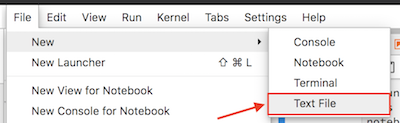This will create a new tab in your JupyterLab window that should look something like that below, a blank slate.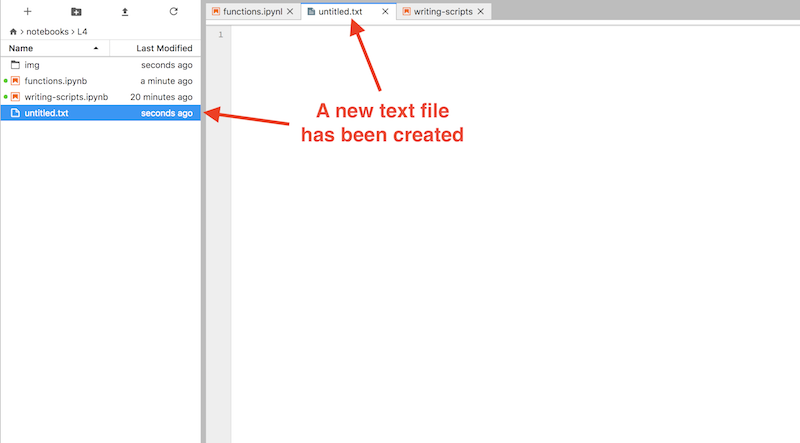Start by copying and pasting the text below into your new text file editor panel.

def celsiusToFahr(tempCelsius):
return 9/5 * tempCelsius + 32


### Saving a text file as a Python file¶

As it turns out, Python scripts are just regular text files with a certain file extension to identify them as source code for Python. In order for our new text file to be detected as a Python source file in JupyterLab we need to rename it to have a .py file extension. You can rename the file by right clicking on the tab titled “untitled.txt” and renaming it as “temp_converter.py”.

Note

Be sure you change the .txt file extension to .py.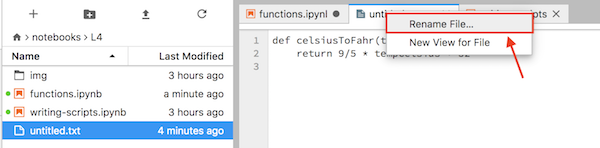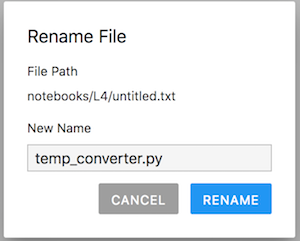If all goes well, you should now see the Python syntax is highlighted in different colors in the JupyterLab editor panel.

Note

Be sure to save your temp_converter.py file after making your changes.

We’ll return later to some best practices for writing script files, but for now let’s continue with how to use our functions saved in the Python file we just created.

Functions such as the ones we just created can also be saved in a script file and called from Jupyter notebooks in JupyterLab. In fact, quite often it is useful to create a dedicated function library for functions that you use frequently, when doing data analysis, for example. Basically this is done by listing useful functions in a single .py file from which you can then import and use them whenever needed.

### Saving functions in a script file¶

Basically, we’ve just seen how to save some functions to a script file. Let’s now add the other functions we had been using to our script. Simply copy and paste the text below into your temp_converter.py file leaving one blank line between each function.

def kelvinsToCelsius(tempKelvins):
return tempKelvins - 273.15

def kelvinsToFahrenheit(tempKelvins):
tempCelsius = kelvinsToCelsius(tempKelvins)
tempFahr = celsiusToFahr(tempCelsius)
return tempFahr


Don’t forget to save your changes!

## Calling functions from a script file¶

Now that we have saved our temperature conversion functions into a script file we can start using them.

### Making sure we’re in the right working directory¶

Hopefully you have saved you temp_converter.py file in the same location as this Jupyter notebook (functions.ipynb). If so, that’s good, but we need to do one more thing to be able to start working with it. We need to change the working directory in JupyterLab to be the one where the temp_converter.py exists.

First, we can check where we are working currently using an IPython magic command called %ls.

:

%ls

__pycache__/           img/                   temp_converter.py
functions.ipynb        modules.ipynb          writing-scripts.ipynb


Your output from %ls probably looks different than that above, but don’t worry. %ls allows us to see the files located in the directory where we are currently working.

#### Binder users¶

If you are using Binder, you might see

L1/  L2/  L3/  L4/  Untitled.ipynb


for example.

#### CSC notebooks users¶

Those using the CSC notebooks might see something like

installations.sh*  Untitled.ipynb  work/


### Changing the working directory¶

Hopefully you’ve seen some output from %ls like that above. Now we need to change into the directory where you’ve saved your temp_converter.py file.

#### Binder users¶

Those using Binder should type the following to change into the directory containing the temp_converter.py file.

%cd L4/


#### CSC notebooks users¶

Those using the CSC notebooks system should type the following to change into the directory containing the temp_converter.py file.

%cd work/notebooks/notebooks/L4/


If all has gone well you should now see temp_converter.py among the files when you type %ls in a Python cell. Try that out below.

:

%ls

__pycache__/           img/                   temp_converter.py
functions.ipynb        modules.ipynb          writing-scripts.ipynb


Now we can continue.

### Importing our script functions¶

Let’s now import our celsiusToFahr() function from the other script by adding a specific import statementain the Python cell below.

:

from temp_converter import celsiusToFahr


### Using our script functions¶

Let’s also use the function so that we can see that it is working.

:

print("The freezing point of water in Fahrenheit is:", celsiusToFahr(0))

The freezing point of water in Fahrenheit is: 32.0


You should get following output:#### Importing multiple functions¶

It is also possible to import more functions at the same time by listing and separating them with a comma.

from my_script import func1, func2, func3


### Importing all functions from a script¶

Sometimes it is useful to import the whole script and all of its functions at once. Let’s use a different import statement and test that all functions work.

:

import temp_converter as tc

:

print("The freezing point of water in Fahrenheit is:", tc.celsiusToFahr(0))

The freezing point of water in Fahrenheit is: 32.0

:

print('Absolute zero in Celsius is:', tc.kelvinsToCelsius(tempKelvins=0))

Absolute zero in Celsius is: -273.15

:

print('Absolute zero in Fahrenheit is:', tc.kelvinsToFahrenheit(tempKelvins=0))

Absolute zero in Fahrenheit is: -459.66999999999996


## Temperature calculator (optional, advanced topic)¶

So far our functions have had only one parameter, but it is also possible to define a function with multiple parameters. Let’s now make a simple tempCalculator function that accepts temperatures in Kelvins and returns either Celsius or Fahrenheit. The new function will have two parameters:

• tempK = The parameter for passing temperature in Kelvin
• convertTo = The parameter that determines whether to output should be in Celsius or in Fahrenheit (using letters C or F accordingly)

### Defining the function¶

Let’s start defining our function by giving it a name and setting the parameters.

def tempCalculator(tempK, convertTo):


Next, we need to add conditional statements that check whether the output temperature is wanted in Celsius or Fahrenheit, and then call corresponding function that was imported from the temp_converter.py file.

def tempCalculator(tempK, convertTo):
# Check if user wants the temperature in Celsius
if convertTo == "C":
# Convert the value to Celsius using the dedicated function for the task that we imported from another script
convertedTemp = kelvinsToCelsius(tempKelvins=tempK)
elif convertTo == "F":
# Convert the value to Fahrenheit using the dedicated function for the task that we imported from another script
convertedTemp = kelvinsToFahrenheit(tempKelvins=tempK)


### Returning the result¶

Next, we need to add a return statement so that our function sends back the value that we are interested in.

def tempCalculator(tempK, convertTo):
# Check if user wants the temperature in Celsius
if convertTo == "C":
# Convert the value to Celsius using the dedicated function for the task that we imported from another script
convertedTemp = kelvinsToCelsius(tempKelvins=tempK)
elif convertTo == "F":
# Convert the value to Fahrenheit using the dedicated function for the task that we imported from another script
convertedTemp = kelvinsToFahrenheit(tempKelvins=tempK)
# Return the result
return convertedTemp


Lastly, as we want to be good programmers, we add a short docstring at the beginning of our function that tells what the function does and how the parameters work.

def tempCalculator(tempK, convertTo):
"""
Function for converting temperature in Kelvins to Celsius or Fahrenheit.

Parameters
----------
tempK: <numerical>
Temperature in Kelvins
convertTo: <str>
Target temperature that can be either Celsius ('C') or Fahrenheit ('F'). Supported values: 'C' | 'F'

Returns
-------
<float>
Converted temperature.
"""

# Check if user wants the temperature in Celsius
if convertTo == "C":
# Convert the value to Celsius using the dedicated function for the task that we imported from another script
convertedTemp = kelvinsToCelsius(tempKelvins=tempK)
elif convertTo == "F":
# Convert the value to Fahrenheit using the dedicated function for the task that we imported from another script
convertedTemp = kelvinsToFahrenheit(tempKelvins=tempK)
# Return the result
return convertedTemp


### Testing the new function¶

That’s it! Now we have a temperature calculator that has a simple control for the user where s/he can change the output by using the convertTo parameter. Now as we added the short docstring in the beginning of the function we can use the help() function in Python to find out how our function should be used. Run the Python cell below and then try running help(tempCalculator).

Attention

Reloading modules from within a Jupyter notebook is a bit of a pain. The easiest option is to restart the IPython kernel by going to Kernel -> Restart kernel…. Note that this will delete all variables currently stored in memory in the Jupyter notebook you’re using, so you may need to re-run some cells.

:

import temp_converter as tc

:

help(tc.tempCalculator)

Help on function tempCalculator in module temp_converter:

tempCalculator(tempK, convertTo)
Function for converting temperature in Kelvins to Celsius or Fahrenheit.

Parameters
----------
tempK: <numerical>
Temperature in Kelvins
convertTo: <str>
Target temperature that can be either Celsius ('C') or Fahrenheit ('F'). Supported values: 'C' | 'F'

Returns
-------
<float>
Converted temperature.



### Using the tempCalculator¶

Let’s use it.

:

tempKelvin = 30

:

temperatureC = tc.tempCalculator(tempK=tempKelvin, convertTo="C")

:

print("Temperature", tempKelvin, "in Kelvins is", temperatureC, "in Celsius")

Temperature 30 in Kelvins is -243.14999999999998 in Celsius